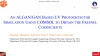# Technical Papers and Presentations

#### An AlGaN/GaN Based UV Photodetector Simulation Using COMSOL® to Obtain the Fresnel Coefficients

B. Uppalapati1, G. Koley1, A. Kota2
1Clemson University, Clemson, SC, USA
2University of Dayton, Dayton, OH, USA
Published in 2020

Over the past two decades, extensive research has been conducted on ultraviolet (UV) photodetectors (PDs) because of their potential applications across various fields including military, medicine, environmental monitoring, and deep space explorations. To fabricate solar-blind UV PDs materials, wide band gap semiconductor materials such as gallium nitride (GaN) and aluminum gallium nitride (AlxGa(1-x)N) are typically used. To design a typical UV PD, so far there are four types of device structures that are widely investigated in the literature. They are Schottky type, metal-semiconductor-metal (MSM) type, p-i-n type, and avalanche type. Each of these device types are realized by using either GaN or AlxGa(1-x)N. In this paper, a novel GaN-AlxGa(1-x)N-GaN vertically stacked cantilever has been proposed to function as a UV PD. The proposed cantilever has been modeled in COMSOL® to compute the Fresnel coefficients such as absorptance, reflectance, and transmittance in the wavelength range of 300 nm to 500 nm by utilizing the Wave Optics Module in COMSOL®. The 3D geometry of the GaN-AlxGa(1-x)N-GaN cantilever is shown in Fig. 1. Instead of simulating the entire 3D geometry, to simplify the problem a 2D longitudinal cross-section at the tip region of the cantilever is used, as shown in Fig. 2. The thickness of the AlxGa(1-x)N and top and bottom GaN layers is 20 nm, 3 nm, and 1 µm, respectively. To simulate the Fresnel coefficients, the required input material property for both GaN and AlxGa(1-x)N is their refractive index. In this model, for the given 2D geometry a linearly polarized electromagnetic wave was launched in the -y axis. It was assumed that the electric (E) and magnetic (H) field components are along z and x axis respectively. As the H-field components are along the x axis, a perfect magnetic conductor (PMC) boundary condition was applied on both sides of the 2D geometry.
Using the wavelength domain study, Fresnel coefficients were computed in the given wavelength range. Simulations are also performed for two different cases. The results obtained from these studies are shown in Figs. 3&4 respectively. The first case is to study the effect of bottom GaN layer thickness variation on the absorption property of the cantilever. The thickness of the bottom GaN layer is varied from 0.1 to 2 µm with a step size of 0.1 µm. From the simulation results it has been observed that as the thickness of the bottom GaN layer increases from 0.1 µm to 0.8 µm, the cantilever absorptance increased monotonically from 74.77% to 86.52% with Al alloy composition remaining fixed at 19%. But for thicknesses > 0.8 μm the absorptance profile remains basically unchanged. We also studied the effect of Al alloy composition in AlxGa(1-x)N on the absorption properties. From the simulation results it has been observed that as the Al% in AlxGa(1-x)N increases from 19% to 78.5%, the cantilever absorptance also increased monotonically. Specifically, at 300 nm wavelength as the Al% is increased from 19% to 78.5%, the absorption percentage is increased from 86.5% to 91% for a GaN thickness of 1.3 µm.